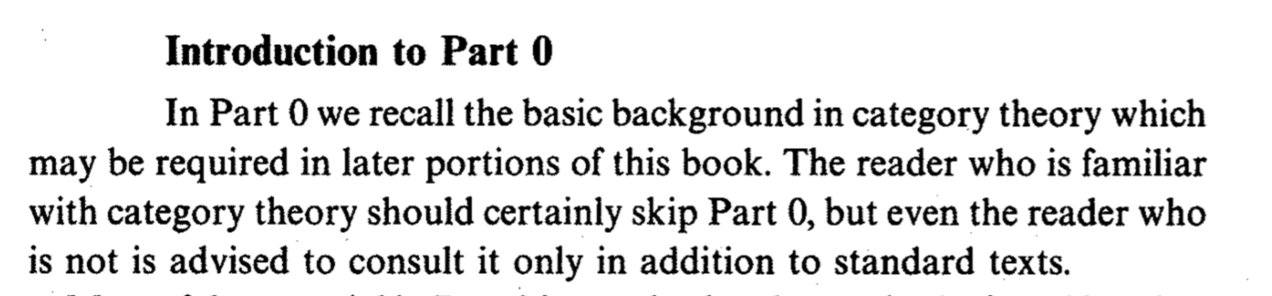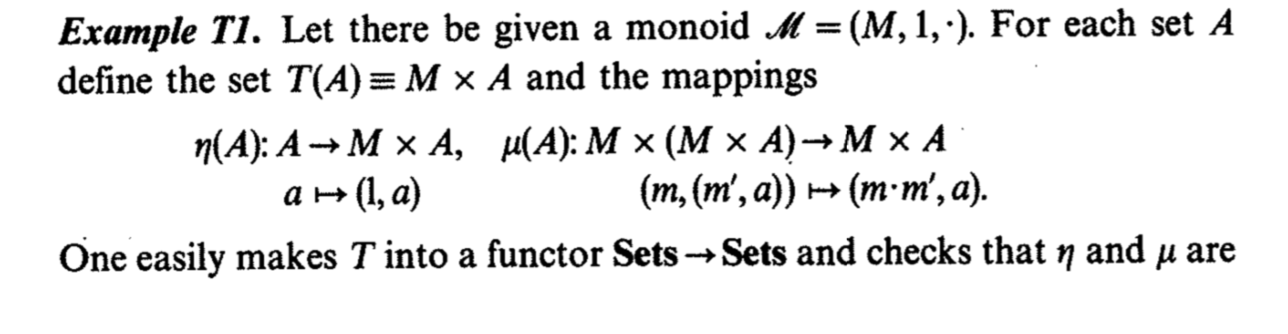# On our Agda experience in Category theory, Hoare logic, Automaton and System F

Menu Menu

Shinji KONO

## In this talk

```    Category in Agda
Automaton in Agda
Hoare Logic in Agda
Continuation based C
Meta computation and Monad
Reflection in Agda

```

## How we study category

To understand Monad in Haskell

implementations of monads are complicated

In Higher order categorical logic## Category

From section 0 of Higher Order Categorical Logic, I found that A translation from this book into Agda is very direct. If M is a monoid and Functor

```    T(A) ≡ M × A

```
is written like this...

```    T : Functor A A
T = record {
FObj = λ a → (Carrier M) × a
; FMap = λ f p → (proj₁ p , f (proj₂ p ))
...

```
(FMap is not mentioned in the book though) The natural transformation η and μ are

## Type is absent in the bookis translated into

```    η = λ a → λ(x : a) → ( ε M , x ) ;
μ a ( m , ( m' , x ) ) = ( m ∙ m' ,  x )

```
We have to fill the skipped type.

```    μ : (a : Obj A  ) → FObj T ( FObj T a ) → Σ (Carrier M) (λ x → a )

```

## What we have done

```   Yoneda lemma
Adjoint functor and solution of Universal problem
Limit from product and equalizer
Completeness of Sets
Freyd adjoint functor theorem

```
category-exercise-in-agda in github

## Automaton

```    record Automaton ( Q : Set ) ( Σ : Set  )
: Set  where
field
δ : Q → Σ → Q
astart : Q
aend : Q → Bool

```
very simple.

```    record NAutomaton ( Q : Set ) ( Σ : Set  )
: Set  where
field
Nδ : Q → Σ → Q → Bool
Nstart : Q → Bool
Nend  :  Q → Bool

```
even NFA.

## Subset construction

```    δconv : { Q : Set } { Σ : Set  } { n  : ℕ }  → FiniteSet Q {n}
→  ( nδ : Q → Σ → Q → Bool ) → (Q → Bool) → Σ → (Q → Bool)
δconv {Q} { Σ} { n} N nδ f i q =  exists N ( λ r → f r ∧ nδ r i q )
subset-construction : { Q : Set } { Σ : Set  } { n  : ℕ }  → FiniteSet Q {n} →
(NAutomaton Q  Σ ) → (Automaton (Q → Bool)  Σ )
subset-construction {Q} { Σ} {n} N NFA = record {
δ = λ q x → δconv N ( Nδ NFA ) q x
;  astart = Nstart NFA
;  aend = exists N ( λ q → f q ∧ Nend NFA q )
}

```

## exists in finteSet

we need finiteness of Q to use exists.

```    record FiniteSet ( Q : Set ) { n : ℕ }
: Set  where
field
...
exists : ( Q → Bool ) → Bool
exists p = ...

```

## Don't use List

```    record NAutomaton'( Q : Set ) ( Σ : Set  )
: Set  where
field
nδ : Q → Σ → List Q
sid : Q  →  ℕ
nstart : Q
nend  : Q → Bool

```
then subset construction becomes mess.

## Proofs in Automaton theory

Recursion is no good in automaton proofs.

For example, correctness of subset construction.

So it is Very difficult we need something.

Something like Hoare Logic.

## Hoare logic

```    module Hoare
(PrimComm : Set) (Cond : Set)
(Axiom : Cond -> PrimComm -> Cond -> Set)
where
data Comm : Set where
PComm : PrimComm -> Comm
Seq   : Comm -> Comm -> Comm
If    : Cond -> Comm -> Comm -> Comm
While : Cond -> Comm -> Comm
data HTProof : Cond -> Comm -> Cond -> Set where
PrimRule : {bPre : Cond} -> {pcm : PrimComm} -> {bPost : Cond} ->
(pr : Axiom bPre pcm bPost) ->
HTProof bPre (PComm pcm) bPost
...

```
Hoare Logic in our mercurial repository

## Program of Hoare logic

```    program : ℕ → Comm
program c10 =
Seq ( PComm (λ env → record env {varn = c10}))
\$ Seq ( PComm (λ env → record env {vari = 0}))
\$ While (λ env → lt zero (varn env ) )
(Seq (PComm (λ env → record env {vari = ((vari env) + 1)} ))
\$ PComm (λ env → record env {varn = ((varn env) - 1)} ))

```

## Continuation based C

An extension of C. Similar to cmiusmius in GHC.

We call __code code gear. The arguments of a code gear are called data gear.

```    __code cbc_next(INTERP i){
__code (*c)(INTERP);
c = CODES[NEXT_OP(i)];
goto c(i);
}
__code cbc_no_op(INTERP i){
goto cbc_next(i);
}

```## data gear (normal C struct)

```    typedef struct interp {
MVMuint16 op;
MVMRegister *reg_base;
...
} INTER,*INTERP;
__code (* CODES[])(INTERP) = {
cbc_no_op,
cbc_no_op,
cbc_gc,
cbc_exit,
};

```

## Implementation of CbC

LLVM version of CbC GCC version of CbC It supports full set of C and its optimization.

Forcing tail call in __code and parameterized goto.

Some bugs, eg. can't handle local variable pointer.

## Intention of CbC

This is not for human, not so easy to read. We have to handle stack and function call directly.

This is intended for machines or AIs.

There are no stack that may contain dynamic or open typing.

Take

```     easy to prove

```
instead of
```     easy to program

```

## type of CbC

I'm getting tired to be asked, "What is the operational semantics of CbC?".

Data gear is a data type, Code gear is a lambda term which has a form of type:

```    gear : in -> (out -> t) -> t

```
which is something like

```   __code gear(in x, __code next(out y)) { out y = ... ; goto next(y); }

```

## while program in agda CbC

```    record Env  : Set where
field
varn : ℕ
vari : ℕ
open Env
whileTest : {l : Level} {t : Set l} -> (c10 : ℕ) → (Code : Env -> t) -> t
whileTest c10 next = next (record {varn = c10 ; vari = 0} )
{-# TERMINATING #-}
whileLoop : {l : Level} {t : Set l} -> Env -> (Code : Env -> t) -> t
whileLoop env next with lt 0 (varn env)
whileLoop env next | false = next env
whileLoop env next | true =
whileLoop (record {varn = (varn env) - 1 ; vari = (vari env) + 1}) next
test1 : Env
test1 = whileTest 10 (λ env → whileLoop env (λ env1 → env1 ))

```

## Proof part

```    gear' : in Pre -> (out Post -> t) -> t

```
Actual Proof

```    whileTest' : {l : Level} {t : Set l}  -> {c10 :  ℕ } → (Code : (env : Env)
-> ((vari env) ≡ 0) /\ ((varn env) ≡ c10) -> t) -> t
whileTest' {_} {_} {c10} next = next env proof2
where
env : Env
env = record {vari = 0 ; varn = c10}
proof2 : ((vari env) ≡ 0) /\ ((varn env) ≡ c10) -- PostCondition
proof2 = record {pi1 = refl ; pi2 = refl}

```

## Actual Proof (cont')

```    {-# TERMINATING #-} --
whileLoop' : {l : Level} {t : Set l} -> (env : Env) -> {c10 :  ℕ }
→ ((varn env) + (vari env) ≡ c10) -> (Code : Env -> t) -> t --  PreCondition(Invaliant)
whileLoop' env proof next with  ( suc zero  ≤? (varn  env) )
whileLoop' env proof next | no p = next env
whileLoop' env {c10} proof next | yes p = whileLoop' env1 (proof3 p ) next
where
env1 = record {varn = (varn  env) - 1 ; vari = (vari env) + 1}
1<0 : 1 ≤ zero → ⊥
1<0 ()
proof3 : (suc zero  ≤ (varn  env))  → varn env1 + vari env1 ≡ c10
proof3 = ...

```

## Actual Proof (cont')

```    -- weakening
conversion1 : {l : Level} {t : Set l } → (env : Env) -> {c10 :  ℕ } →
((vari env) ≡ 0) /\ ((varn env) ≡ c10)
-> (Code : (env1 : Env) -> (varn env1 + vari env1 ≡ c10) -> t) -> t
proofGears : {c10 :  ℕ } → Set
proofGears {c10} = whileTest' {_} {_} {c10} (λ n p1 →
conversion1 n p1 (λ n1 p2 → whileLoop' n1 p2 (λ n2 →  ( vari n2 ≡ c10 ))))

```

## Meta computation on gear

```    gear : in -> (out -> t) -> t

```
for some Monad M,

```    gearM : in  -> (out -> M t) -> M t

```
gearM and gear is acutely the same.## Meta computation and Monad

There should only one Monad in a computer, that is the operating system.

Basic part of a program should be simple, but error handling or resource management make it complex.

Leave the complex part in meta computation.

Give two implementations over an interface,

```   one for efficient and fast execution
one for provability

```
An interface gives basic property of a library call (as an agda record).

## What we are doing ...

Rewriting x.v6 OS using CbC and CbC Agda.

CbC has GCC and LLVM implementations.

Writing some proof template with basic libraries.

Shinji KONO / Tue Mar 19 09:27:20 2019